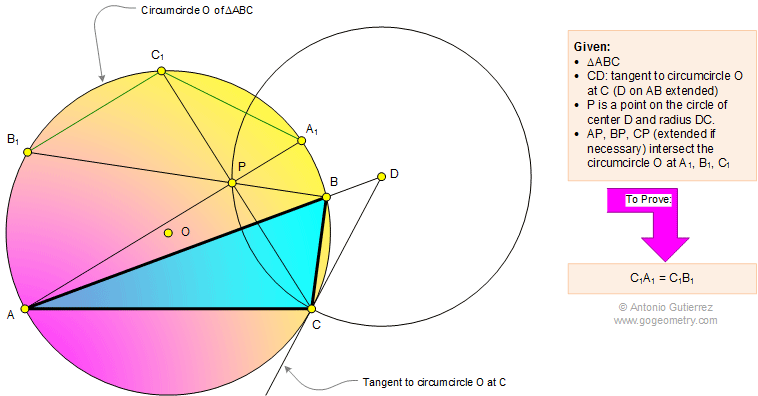# Geometry Problem 1107: Triangle, Circumcircle, Circle, Radius, Tangent, Chord, Congruence. Level: High School, SAT Prep, College Geometry

< PREVIOUS PROBLEM  |  NEXT PROBLEM >

 In a triangle ABC (see the figure below) CD is tangent to circumcircle O at C (D on AB extended) and P is a point on the circle of center D and radius DC. AP, BP, and CP (extended if necessary) intersect the circumcircle O at A1, B1, and C1, respectively. Prove that C1A1 = C1B1.Home | Search | Geometry | Problems | All Problems | Open Problems | Visual Index | 1101-1110 | Triangle | Circle | Tangent Line | Circumcircle | Congruence | Email | Post a comment or solutionLast updated: Apr 4, 2015, by Antonio Gutierrez